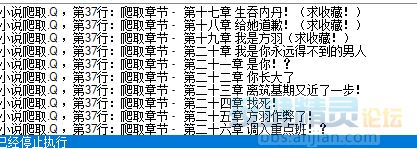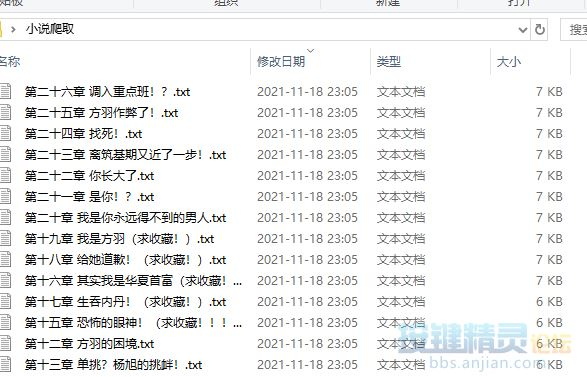1#1. 网页 = "https://www.xbiquge.la" : 完整网页 = 网页 & "/32/32522/"
2. 原网页源码 = 网页_获取网页源文件(完整网页)
4. If Plugin.File.IsFileExist(保存位置) = false Then
5. Call Plugin.File.CreateFolder(保存位置)
6. TracePrint "创建小说文件夹"
7. End If
9. 开始位置 = 1
10. If 原网页源码 <> "" Then
11. Do
12. 内容 = 取中间文本(原网页源码, "<dd><a href='", "' >第", 开始位置,1)
13. 内容 = Replace(内容, "<dd><a href='", "")
14. If 内容 <> "" Then
15. // TracePrint "网址" & 网页 & 内容
16. 获取章节正文 网页 & 内容
17. Else
18. TracePrint "内容为空,停止" & time : Exit Do
19. End If
20. Loop
21. End If
22. Function 获取章节正文(网址)
23. For 20
24. 没处理网页源码 = 网页_获取网页源文件(网址)
26. If 没处理网页源码 <> "" Then
27. 章节 = 取中间文本(没处理网页源码, " <h1>全部章节", "</h1>", 1,0)
28. 章节 = Replace(章节, "<h1>", "") : 章节 = Replace(章节, "全部章节", "")
29. 文章内容 = 取中间文本(没处理网页源码, " ", "<p><a href=", 1,0)
30. 文章内容 = Replace(文章内容, "<br /> ", "") '删除正文中的多余符号
31. 文章内容 = Replace(文章内容, "<br />", "") '删除正文中的多余符号
32. 文章内容 = Replace(文章内容, " ", "") '删除正文中的多余符号
33. If 文章内容 <> "" Then
34. Plugin.File.WriteFileEx 保存位置 & 章节 & ".txt", 章节
35. Plugin.File.WriteFileEx 保存位置 & 章节 & ".txt", 文章内容
36. TracePrint "爬取章节 - " & 章节 : Exit Function
37. End If
38. End If
39. Next
40. TracePrint "内容为空,爬取结束 " & 网址 : ExitScript
41. End Function
42. Function 取中间文本(原文本, 左边文本, 右边文本, 左边位置,赋值1)
43. Dim 返回长度, 开始, 结束
44. 取中间文本=""
45. 开始 = InStr(左边位置, 原文本, 左边文本, 1)
46. If 开始 > 0 Then
47. 结束 = InStr(开始, 原文本, 右边文本, 1)
48. If 结束 > 开始 Then
49. 返回长度 = 结束 - 开始
50. 取中间文本 = mid(原文本, 开始, 返回长度)
51. If 赋值1 = 1 Then 开始位置 = 结束
52. Else
53. TracePrint "取中间文本错误,开始" & 开始 & "结束" & 结束
54. End If
55. End If
56. End Function
57. Function 网页_获取网页源文件(网址)
58. '此函数可以模拟成真实访问
59. Set xmlHttp = CreateObject("Microsoft.XMLHTTP")
60. xmlHttp.Open "Get", 网址, False
61. xmlHttp.Send
62. 网页_获取网页源文件 = xmlHttp.ResponseText
63. Set xmlHttp = Nothing
64. End Function

===================效果图========================